# Cole Striler

# Importing Libraries
import pandas as pd
pd.set_option("display.max_columns", None)
import numpy as np
import seaborn as sns
import matplotlib.pyplot as plt

from sklearn.preprocessing import LabelEncoder
from sklearn.model_selection import train_test_split
from sklearn.linear_model import LogisticRegression
from sklearn.ensemble import RandomForestClassifier
from sklearn.tree import DecisionTreeClassifier
from sklearn.metrics import confusion_matrix, classification_report, accuracy_score
from sklearn.model_selection import cross_val_score
from xgboost import XGBClassifier, plot_importance

# Import Warnings Filter
from warnings import simplefilter
# Ignore all Future Warnings
simplefilter(action='ignore', category=FutureWarning)
import warnings
warnings.filterwarnings('ignore')


# John Deere - Analytics Case Study

# Loading / Creating DataFrames
wilderness_1 = data[data['Wilderness_Area_1'] == 1]
wilderness_2 = data[data['Wilderness_Area_2'] == 1]
wilderness_3 = data[data['Wilderness_Area_3'] == 1]
wilderness_4 = data[data['Wilderness_Area_4'] == 1]
soil = data.iloc[:, 15:55]

print("DataFrames:     Shape:")
print("- data:         ", data.shape)
print("- wilderness_1: ", wilderness_1.shape)
print("- wilderness_2: ", wilderness_2.shape)
print("- wilderness_3: ", wilderness_3.shape)
print("- wilderness_4: ", wilderness_4.shape)
print("- soil:         ", soil.shape)

DataFrames:     Shape:
- data:          (581012, 55)
- wilderness_1:  (260796, 55)
- wilderness_2:  (29884, 55)
- wilderness_3:  (253364, 55)
- wilderness_4:  (36968, 55)
- soil:          (581012, 40)

# Summary Statistics (only showing first 3 variables)
data.iloc[:, :3].describe()

Elevation Aspect Slope
count 581012.000000 581012.000000 581012.000000
mean 2959.365301 155.656807 14.103704
std 279.984734 111.913721 7.488242
min 1859.000000 0.000000 0.000000
25% 2809.000000 58.000000 9.000000
50% 2996.000000 127.000000 13.000000
75% 3163.000000 260.000000 18.000000
max 3858.000000 360.000000 66.000000
# Dimensions of the Dataset
data.shape

(581012, 55)

# EDA

Since the goal of this project is to predict Cover Type, I want to first look at the Cover_Type variable.

# Plotting Cover Types
sns.countplot(x='Cover_Type', data=data)
plt.title("Frequency of Cover Types")
plt.show()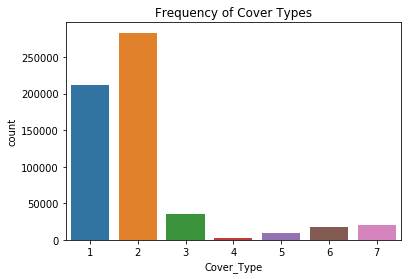As the above plot shows, Cover Types 1 and 2 are significantly more common than Cover Types 3-7. I also want to take a look at the other columns in the dataset to get a better understanding of what I'm working with and to see if anything stands out. In the back of my mind, I'm starting to think about which variables may be able to help us predict a tree's Cover_Type.

# Plotting Histograms
data.iloc[:, :11].hist(figsize = (15,10))
plt.show()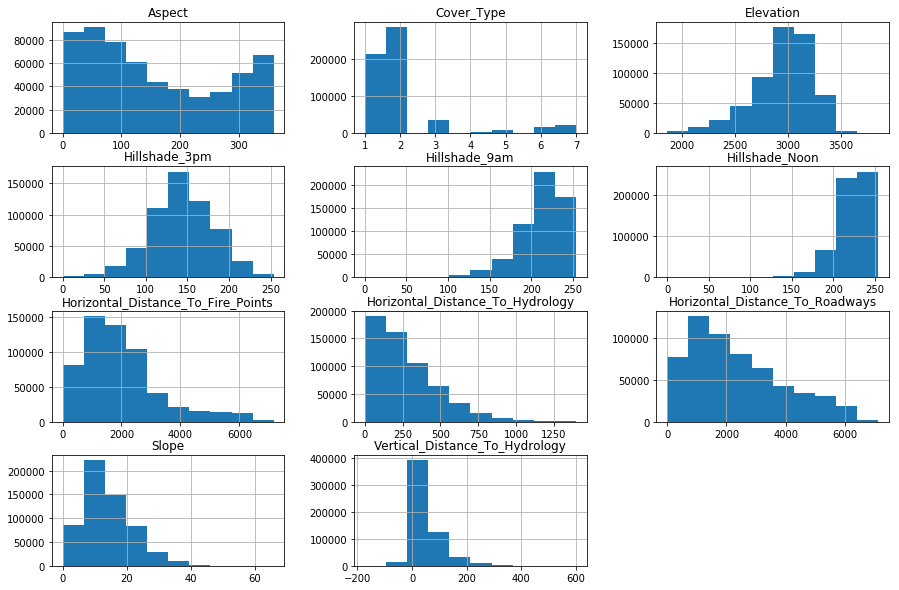Nothing significant stands out from looking at these distributions. As of now, my intuition tells me that each of the 11 variables above may be good variables to include in my classification model. I also want to take a look at the different soil types. I think soil could be a great indicator as to which Cover Type a tree might be.

# Plotting Soil Types
soil.sum().plot(kind="bar", figsize=(15,6))
plt.title("Frequency of Each Soil Type")
plt.show()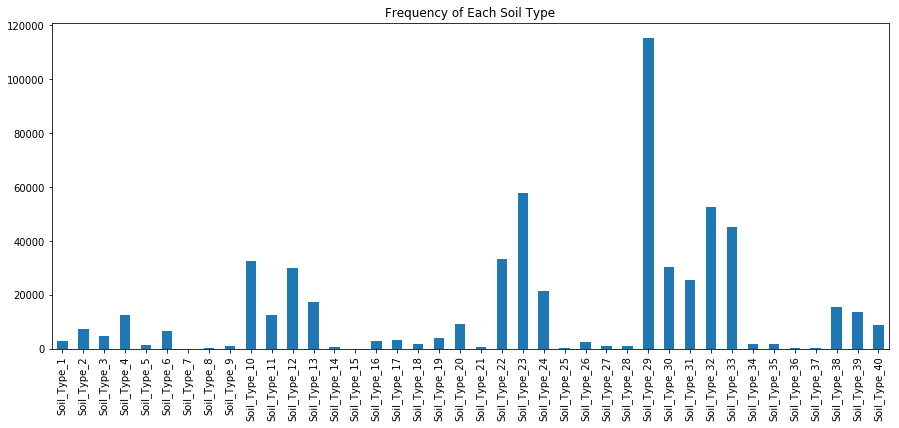This bar plot shows that soil #29 is by far the most common among the trees in our dataset. Other soils, like #7, #15, and #37, occur much less often. Perhaps rare soil types might occur more with rare Cover Types. As of now, I believe soil type could be a good variable to help us predict a tree's Cover Type. Now, I want to take a look at the different wildernesses to see if certain Cover Types are more common in certain regions.

# Generates Wilderness Subplots
fig, ax = plt.subplots(2,2, figsize=(9,9))

sns.countplot(x='Cover_Type', data=wilderness_1, ax=ax[0,0])
ax[0,0].set_title(f"Wilderness 1", size=20)
ax[0,0].set_ylabel('Count')
ax[0,0].set_xlabel('Cover Type')

sns.countplot(x='Cover_Type', data=wilderness_2, ax=ax[0,1])
ax[0,1].set_title(f"Wilderness 2", size=20)
ax[0,1].set_ylabel('Count')
ax[0,1].set_xlabel('Cover Type')

sns.countplot(x='Cover_Type', data=wilderness_3, ax=ax[1,0])
ax[1,0].set_title(f"Wilderness 3", size=20)
ax[1,0].set_ylabel('Count')
ax[1,0].set_xlabel('Cover Type')

sns.countplot(x='Cover_Type', data=wilderness_4, ax=ax[1,1])
ax[1,1].set_title(f"Wilderness 4", size=20)
ax[1,1].set_ylabel('Count')
ax[1,1].set_xlabel('Cover Type')

plt.suptitle(f"Cover Type Counts by Wilderness Number", size=20)
plt.show()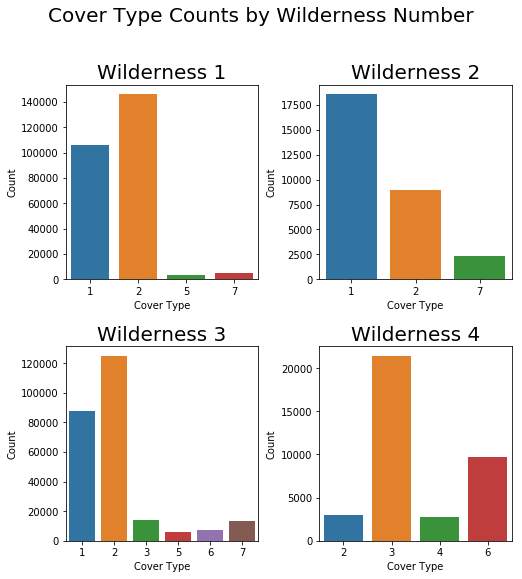These plots show that different wildernesses do in fact have different ratios of Cover Types. In fact, neither of the four wildernesses include trees from all seven cover types. This leads me to believe that wilderness will also be a good indicator of a tree's Cover Type.

As of now, I haven't found any variables that I'd want to exclude from the model. One thing that I want to look at is the correlation between variables to see if there may be any issues with multi-collinearity.

# Generates Correlation Heatmap
corr = data.iloc[:, :10].corr()
plt.figure(figsize=(10,10))
ax = sns.heatmap(
corr,
vmin=-1, vmax=1, center=0,
cmap=sns.diverging_palette(20, 220, n=200),
square=True
)
ax.set_xticklabels(
ax.get_xticklabels(),
rotation=45,
horizontalalignment='right'
);
plt.title("Correlation Between Variables")
plt.show()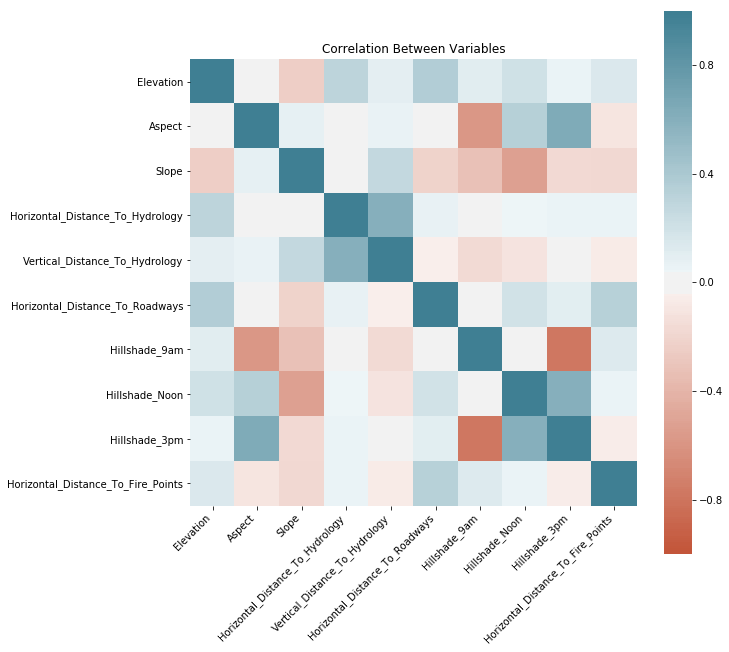This correlation heatmap shows that there is in fact strong negative correlation between the variables Hillshade_3pm and Hillshade_9am. This doesn't seem like a huge issue for the scope of this project, but it is something that might be worth paying attention to in the future.

Now I want to directly compare a few variables with Cover_Type to get a better idea of their relationships. I start by taking a random sample of the dataset (plotting the entire dataset causes major overfitting). In the first scatter plot, I look at Elevation against Cover_Type. In the second plot, I look at the mean Elevation of every Cover_Type.

# Plotting Elevation & Cover Types
plt.figure(figsize=(15,7))
sns.swarmplot(x='Cover_Type', y='Elevation', data=data.sample(2000))
plt.title("Comparing Elevations of Cover Types")
plt.show()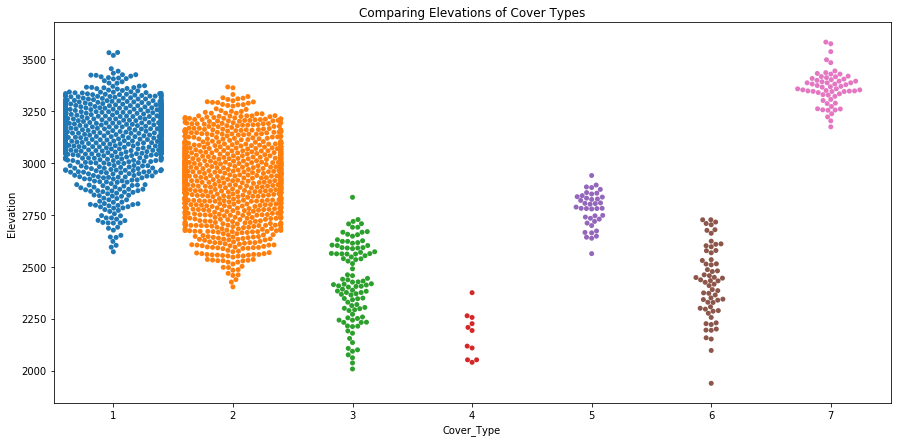# Plotting Means
plt.figure(figsize=(7,5))
data.groupby("Cover_Type").mean()["Elevation"].plot(kind='bar')
plt.title("Mean Elevations of Cover Types")
plt.show()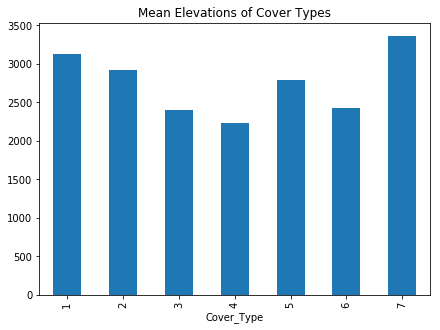Based on the results of these plots, I believe Elevation will be a good variable to help us predict Cover Type. I also think that some of the distance variables could be interesting to look at. Below, I do a similar comparison, this time looking at Horizontal_Distance_To_Roadways against Cover_Type.

# Plotting Horizontal Distance to Roadways & Cover Types
plt.figure(figsize=(15,7))
plt.title("Comparing Distance to Roadways of Cover Types")
plt.show()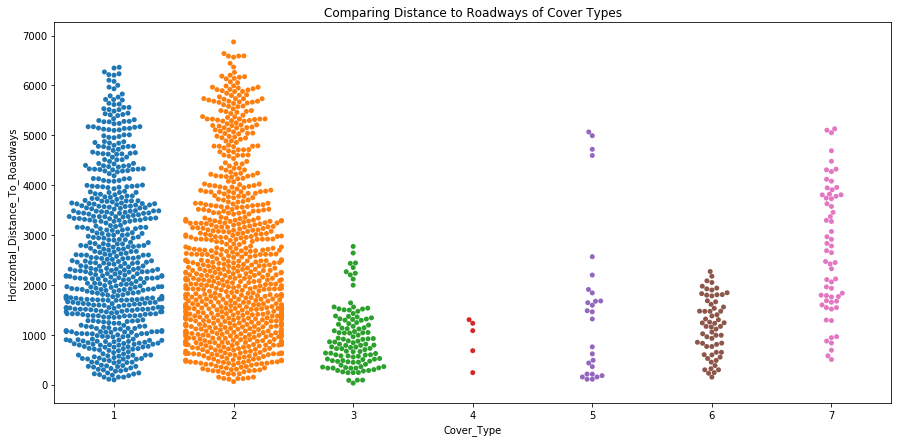# Plotting Means
plt.figure(figsize=(7,5))
plt.title("Mean Distances to Roadways of Cover Types")
plt.show()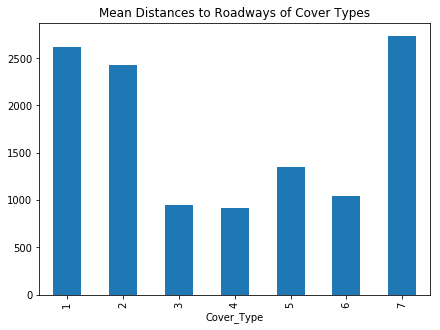One last thing I want to look at are the relationships between the different distance columns. Below shows a scatterplot, a histogram, and a KDE plot for every pair of distance variables.

# Plotting Distance Variables
columns = ['Horizontal_Distance_To_Hydrology','Vertical_Distance_To_Hydrology',
g = sns.PairGrid(data.loc[:, columns].sample(1000), palette='coolwarm')
g = g.map_diag(plt.hist)
g = g.map_upper(plt.scatter, linewidths=1, edgecolor='w', s=40)
g = g.map_lower(sns.kdeplot, cmap='coolwarm')
plt.show()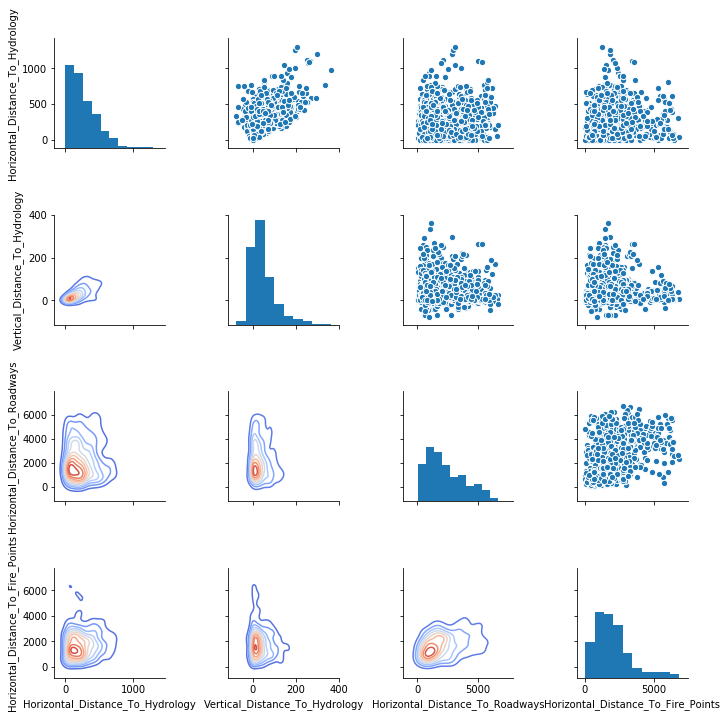Nothing major stands out here, but it gives us a good idea of how the different distances are related.

Based on the above EDA, I don't have strong enough evidence to exclude any of the given variables from the model. If there were a ton of columns, I would be concerned about overfitting the model. I don't think we will run into overfitting issues with only 55 columns, expecially since the number of rows is quite large (581,012). Therefore, I will use every column from the original dataset in the design matrix.

### A few insights that I learned from the EDA process:

• The distribution of Cover Types is not uniform
• Certain soil types are more common than others
• Elevation might be a strong indicator as to which Cover Type a tree might be
• There may be some correlated variables in the dataset
• Every wilderness excludes some Cover Types

# Classification Models

# Splitting Test/Train Datasets
X = data.drop('Cover_Type', axis=1)
y = data['Cover_Type']

X_train, X_test, y_train, y_test = train_test_split(X, y, test_size=0.3, random_state=52)

# Helper Function for Creating Models
keys = []
scores = []

def predict(model, name):
mod = model
mod.fit(X_train, y_train)
pred = mod.predict(X_test)
print("Confusion Matrix:")
print(confusion_matrix(y_test, pred))
print()
print("Classification Report:")
print(classification_report(y_test, pred))
acc = accuracy_score(y_test, pred)
print("Model Accuracy: "+ str(acc))
print('\n' + '\n')
keys.append(name)
scores.append(acc)


### Logistic Regression

The first classification model that comes to mind is the Logistic Regression model. Even though it was designed for binomial classification, it can be used in the multiclass case. I'm really only using this model as a baseline for comparison with other models. I don't think it will give me the best predictions.

# Logistic Regression
predict(LogisticRegression(), "Logistic Regression")

Confusion Matrix:
[[43054 19172    34     0     0     0  1157]
[15034 68283  1598     3     0   129   134]
[   20  1235  9158    32     0   223     2]
[    0     0   668   111     0    27     0]
[   73  2535   189     0     0     5     0]
[    3  1974  3027     5     0   233     0]
[ 3108    36    24     0     0     0  3018]]

Classification Report:
precision    recall  f1-score   support

1       0.70      0.68      0.69     63417
2       0.73      0.80      0.77     85181
3       0.62      0.86      0.72     10670
4       0.74      0.14      0.23       806
5       0.00      0.00      0.00      2802
6       0.38      0.04      0.08      5242
7       0.70      0.49      0.58      6186

micro avg       0.71      0.71      0.71    174304
macro avg       0.55      0.43      0.44    174304
weighted avg       0.69      0.71      0.69    174304

Model Accuracy: 0.7105803653387186



### Decision Tree

I also want to use a non-linear model to give some predictions so I will move to tree based learning algorithm. Tree based algorithms are typically more accurate and are easy to interpret. The first tree based algorithm that I will use is a Decision Tree.

# Decision Tree
predict(DecisionTreeClassifier(), "Decision Tree")

Confusion Matrix:
[[59285  3747     2     0    66    10   307]
[ 3743 80561   237     3   414   186    37]
[    2   244  9881    99    27   417     0]
[    0     0   104   666     0    36     0]
[   53   404    28     0  2302    13     2]
[   14   179   436    27     6  4580     0]
[  318    52     0     0     0     0  5816]]

Classification Report:
precision    recall  f1-score   support

1       0.93      0.93      0.93     63417
2       0.95      0.95      0.95     85181
3       0.92      0.93      0.93     10670
4       0.84      0.83      0.83       806
5       0.82      0.82      0.82      2802
6       0.87      0.87      0.87      5242
7       0.94      0.94      0.94      6186

micro avg       0.94      0.94      0.94    174304
macro avg       0.90      0.90      0.90    174304
weighted avg       0.94      0.94      0.94    174304

Model Accuracy: 0.9356698641454011



### Random Forest

The next learning algorithm I use is a Random Forest. I chose to use a Random Forest because of it's flexibility to new problems and ease of use.

# Random Forest
predict(RandomForestClassifier(n_estimators=300, random_state=52), "Random Forest")

Confusion Matrix:
[[59728  3507     2     0    16     4   160]
[ 1887 82863   169     3   122   118    19]
[    3   154 10257    37     7   212     0]
[    0     0   103   679     0    24     0]
[   42   593    28     0  2126    13     0]
[    3   145   338    23     4  4729     0]
[  293    32     0     0     0     0  5861]]

Classification Report:
precision    recall  f1-score   support

1       0.96      0.94      0.95     63417
2       0.95      0.97      0.96     85181
3       0.94      0.96      0.95     10670
4       0.92      0.84      0.88       806
5       0.93      0.76      0.84      2802
6       0.93      0.90      0.91      5242
7       0.97      0.95      0.96      6186

micro avg       0.95      0.95      0.95    174304
macro avg       0.94      0.90      0.92    174304
weighted avg       0.95      0.95      0.95    174304

Model Accuracy: 0.9537532127776758


# Printing Results
table = pd.DataFrame({'model':keys, 'accuracy score':scores})
print(table)

                 model  accuracy score
0  Logistic Regression        0.710580
1        Decision Tree        0.935670
2        Random Forest        0.953753

# Exploring the Number of Estimators in the Random Forest
score = []
est = []
estimators = [1, 10, 50, 100, 200, 300, 400, 500]
for e in estimators:
rfc1 = RandomForestClassifier(n_estimators=e, random_state=52)
pred1 = rfc1.fit(X_train, y_train).predict(X_test)
accuracy = accuracy_score(y_test, pred1)
score.append(accuracy)
est.append(e)
plot = sns.pointplot(x=est, y=score)
plot.set(xlabel='Number of estimators', ylabel='Accuracy',
title='Accuracy score of RFC per # of estimators')
plt.show()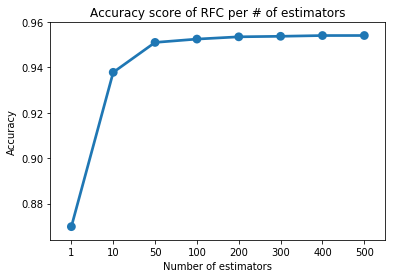I chose to use 300 estimators in the Random Forest because of the results in the above point plot. As we can see, the accuracy of the model stops noticeably increasing at around 200-300 estimators.

# EGB Model
xgb = XGBClassifier(seed=52)
pred = xgb.fit(X_train, y_train).predict(X_test)
print(confusion_matrix(y_test, pred))
print(classification_report(y_test, pred))
print("accuracy is "+ str(accuracy_score(y_test, pred)))

[[46077 16664     8     0    12     7   649]
[13983 70120   722     6    85   250    15]
[    0  1326  8993    81     0   270     0]
[    0     4   296   490     0    16     0]
[    8  2420    81     0   293     0     0]
[    0  1596  3042    34     0   570     0]
[ 2858    15     0     0     0     0  3313]]
precision    recall  f1-score   support

1       0.73      0.73      0.73     63417
2       0.76      0.82      0.79     85181
3       0.68      0.84      0.76     10670
4       0.80      0.61      0.69       806
5       0.75      0.10      0.18      2802
6       0.51      0.11      0.18      5242
7       0.83      0.54      0.65      6186

micro avg       0.74      0.74      0.74    174304
macro avg       0.73      0.54      0.57    174304
weighted avg       0.74      0.74      0.73    174304

accuracy is 0.7449972461905636

# Plotting Variable Importance
plot_importance(xgb)
plt.rcParams['figure.figsize']=(10,20)
plt.show()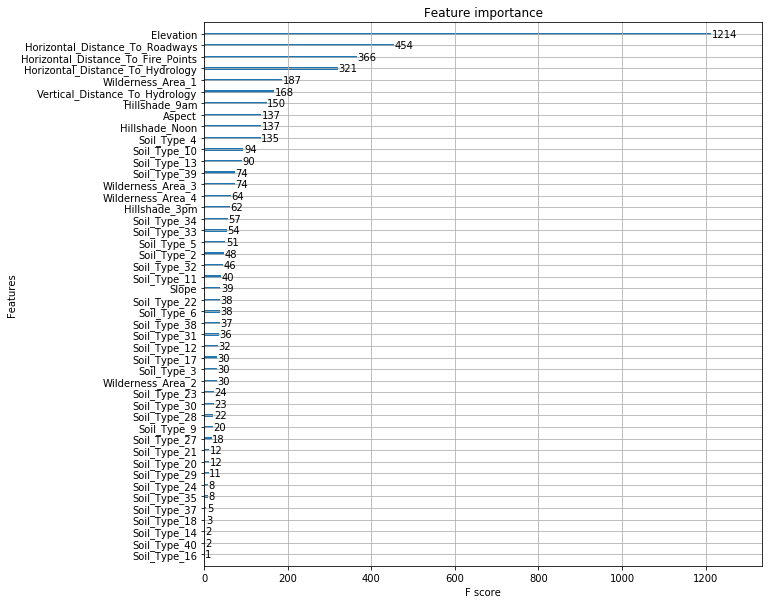The accuracy of the EGB model above can be improved by tuning the hyper-parameters, but I will not go into that for this project. The above importance plot is pretty cool to look at. It confirms my original hypothesis that Elevation is a great variable to predict Cover Type. It also showed the distance variables to be pretty strong variables, which was a little less clear from the EDA.

# Final Results

Of the four models I chose to use, the Random Forest gave the best predictions with 95.4% accuracy. If more time were allotted, I would spend more time looking at correlated variables and fine-tuning the EGB model because these are two things that I believe could improve the accuracy of the predictions.